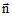# Definition of regions for a complete computation

## Introduction

The complete computation is a computation carried out under the following conditions:

• computation is accomplished with taking into account of the current density in the structure
• the structure introduced in the electrolyte is represented by means of thin regions and boundary conditions.

## Volume region

Volume regions are gathered in the table below.

A volume region…

is a material region which enables the modeling…

electrolytic conducting of a conducting medium (resistivity ρ)
metal conducting of a conducting medium (resistivity ρ)
inactive of an insulating medium

## Face region

Face regions are gathered in the table below.

A thin region… = material region + BC

metal conducting (without contact with the electrolyte)

conducting medium (resistivity ρ)

tangent electric field on both sides

metal conducting with electrochemical interface

conducting medium (resistivity ρ)

condition at the interface “electrolyte – cathode” via polarization law

metal conducting with imposed normal current density

conducting medium (resistivity ρ)

a normal current density* (varying formula / spatial formula) (active anode)

metal conducting insulated from the electrolyte on both sides conducting medium (resistivity ρ)

tangent electric field on both sides

Note: * The normalis directed from the face region towards the electrolyte.

## Line region

Line regions are gathered in the table below.

A filiform region…

is a material region which enables the modeling…

conducting region (uniform current density through the section) of a conducting medium (resistivity ρ)

## Point region

Point regions are gathered in the table below.

A point region… is a BC that enables one to impose…
imposed potential a potential in a point

## Reference of electric potential:

As a problem of electrolysis has in the majority of cases an electrochemical interface, with a polarization law for the 1st computation it is not necessary to impose the electric potential equal to zero to the volume regions of the electrolyte.

For the 2nd computation, you must impose an electric potential equal to zero at a point of the metal conducting regions, the electric potential of the 2nd computation being independent from the electric potential of the 1st calculation.